/pandas 0.23

# pandas.DataFrame.boxplot

`DataFrame.boxplot(column=None, by=None, ax=None, fontsize=None, rot=0, grid=True, figsize=None, layout=None, return_type=None, **kwds)` [source]

Make a box plot from DataFrame columns.

Make a box-and-whisker plot from DataFrame columns, optionally grouped by some other columns. A box plot is a method for graphically depicting groups of numerical data through their quartiles. The box extends from the Q1 to Q3 quartile values of the data, with a line at the median (Q2). The whiskers extend from the edges of box to show the range of the data. The position of the whiskers is set by default to `1.5 * IQR (IQR = Q3 - Q1)` from the edges of the box. Outlier points are those past the end of the whiskers.

For further details see Wikipedia’s entry for boxplot.

Parameters: column : str or list of str, optional Column name or list of names, or vector. Can be any valid input to `pandas.DataFrame.groupby()`. by : str or array-like, optional Column in the DataFrame to `pandas.DataFrame.groupby()`. One box-plot will be done per value of columns in `by`. ax : object of class matplotlib.axes.Axes, optional The matplotlib axes to be used by boxplot. fontsize : float or str Tick label font size in points or as a string (e.g., `large`). rot : int or float, default 0 The rotation angle of labels (in degrees) with respect to the screen coordinate sytem. grid : boolean, default True Setting this to True will show the grid. figsize : A tuple (width, height) in inches The size of the figure to create in matplotlib. layout : tuple (rows, columns), optional For example, (3, 5) will display the subplots using 3 columns and 5 rows, starting from the top-left. return_type : {‘axes’, ‘dict’, ‘both’} or None, default ‘axes’ The kind of object to return. The default is `axes`. ‘axes’ returns the matplotlib axes the boxplot is drawn on. ‘dict’ returns a dictionary whose values are the matplotlib Lines of the boxplot. ‘both’ returns a namedtuple with the axes and dict. when grouping with `by`, a Series mapping columns to `return_type` is returned. If `return_type` is `None`, a NumPy array of axes with the same shape as `layout` is returned. **kwds All other plotting keyword arguments to be passed to `matplotlib.pyplot.boxplot()`. result : The return type depends on the `return_type` parameter: ‘axes’ : object of class matplotlib.axes.Axes ‘dict’ : dict of matplotlib.lines.Line2D objects ‘both’ : a nametuple with strucure (ax, lines) For data grouped with `by`: `Series` `array` (for `return_type = None`)

See also

`Series.plot.hist`
Make a histogram.
`matplotlib.pyplot.boxplot`
Matplotlib equivalent plot.

#### Notes

Use `return_type='dict'` when you want to tweak the appearance of the lines after plotting. In this case a dict containing the Lines making up the boxes, caps, fliers, medians, and whiskers is returned.

#### Examples

Boxplots can be created for every column in the dataframe by `df.boxplot()` or indicating the columns to be used:

```>>> np.random.seed(1234)
>>> df = pd.DataFrame(np.random.randn(10,4),
...                   columns=['Col1', 'Col2', 'Col3', 'Col4'])
>>> boxplot = df.boxplot(column=['Col1', 'Col2', 'Col3'])
```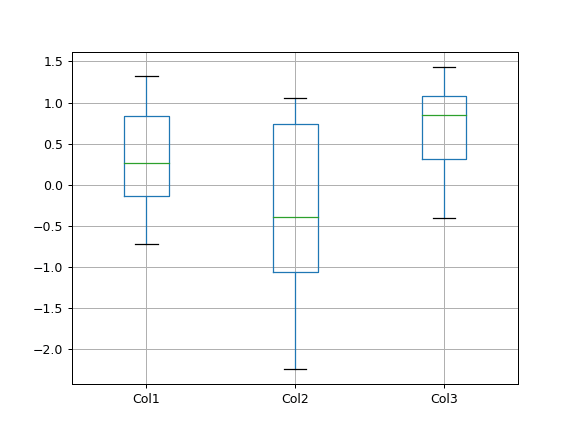Boxplots of variables distributions grouped by the values of a third variable can be created using the option `by`. For instance:

```>>> df = pd.DataFrame(np.random.randn(10, 2),
...                   columns=['Col1', 'Col2'])
>>> df['X'] = pd.Series(['A', 'A', 'A', 'A', 'A',
...                      'B', 'B', 'B', 'B', 'B'])
>>> boxplot = df.boxplot(by='X')
```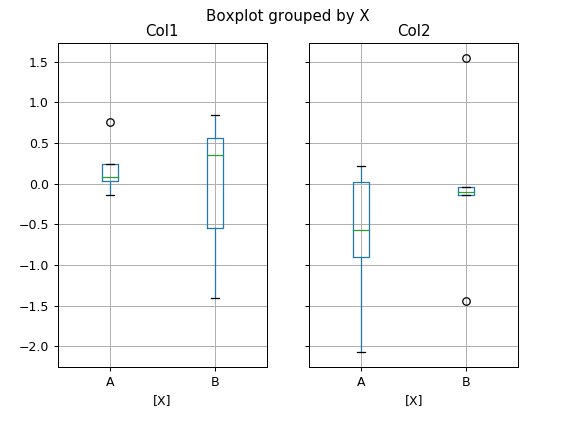A list of strings (i.e. `['X', 'Y']`) can be passed to boxplot in order to group the data by combination of the variables in the x-axis:

```>>> df = pd.DataFrame(np.random.randn(10,3),
...                   columns=['Col1', 'Col2', 'Col3'])
>>> df['X'] = pd.Series(['A', 'A', 'A', 'A', 'A',
...                      'B', 'B', 'B', 'B', 'B'])
>>> df['Y'] = pd.Series(['A', 'B', 'A', 'B', 'A',
...                      'B', 'A', 'B', 'A', 'B'])
>>> boxplot = df.boxplot(column=['Col1', 'Col2'], by=['X', 'Y'])
```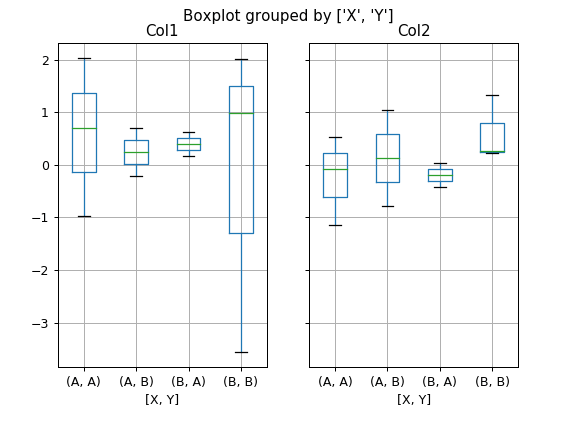The layout of boxplot can be adjusted giving a tuple to `layout`:

```>>> boxplot = df.boxplot(column=['Col1', 'Col2'], by='X',
...                      layout=(2, 1))
```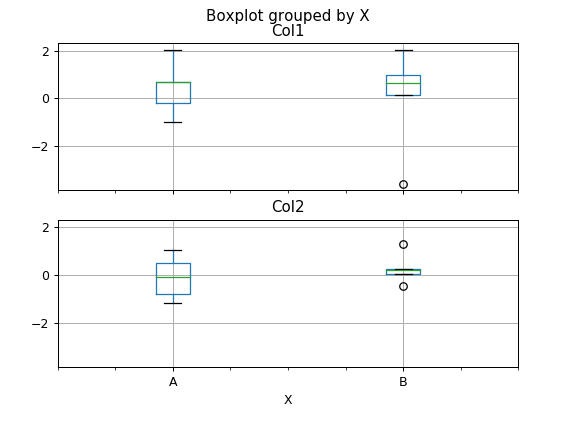Additional formatting can be done to the boxplot, like suppressing the grid (`grid=False`), rotating the labels in the x-axis (i.e. `rot=45`) or changing the fontsize (i.e. `fontsize=15`):

```>>> boxplot = df.boxplot(grid=False, rot=45, fontsize=15)
```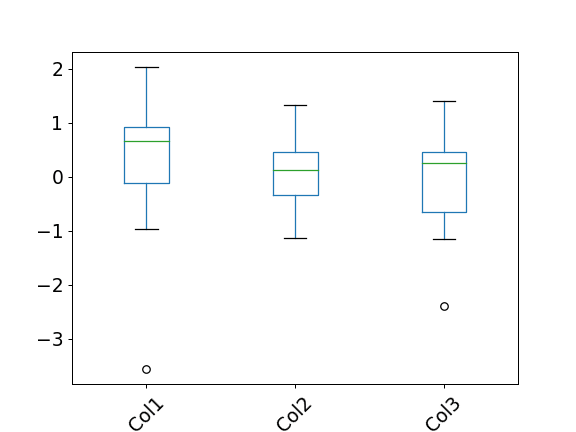The parameter `return_type` can be used to select the type of element returned by `boxplot`. When `return_type='axes'` is selected, the matplotlib axes on which the boxplot is drawn are returned:

```>>> boxplot = df.boxplot(column=['Col1','Col2'], return_type='axes')
>>> type(boxplot)
<class 'matplotlib.axes._subplots.AxesSubplot'>
```

When grouping with `by`, a Series mapping columns to `return_type` is returned:

```>>> boxplot = df.boxplot(column=['Col1', 'Col2'], by='X',
...                      return_type='axes')
>>> type(boxplot)
<class 'pandas.core.series.Series'>
```

If `return_type` is `None`, a NumPy array of axes with the same shape as `layout` is returned:

```>>> boxplot =  df.boxplot(column=['Col1', 'Col2'], by='X',
...                       return_type=None)
>>> type(boxplot)
<class 'numpy.ndarray'>
```

© 2008–2012, AQR Capital Management, LLC, Lambda Foundry, Inc. and PyData Development Team
Licensed under the 3-clause BSD License.
http://pandas.pydata.org/pandas-docs/version/0.23.4/generated/pandas.DataFrame.boxplot.html